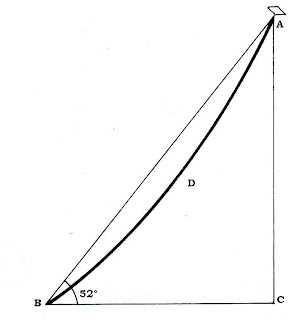### Measuring alttitude - Hauteur du vol

< version française >The satisfaction if determining the altitude of a kite is as great as the challenge that it represents. Calculating height is not a simple operation without a theodolite or an electronic rangefinder, but it can be done.
Referring to the right-angled triangle ABC below, a number of enthusiasts use commercial rangefinders to establish the distance between themselves and their kite BA, while others measure the flying line as it is taken off the reel BDA. This is done in order to establish the length of the hypotenuse BA of the right-angled triangle ABC. Assuming BC to be horizontal, the angle ABC is measured (52°) and its logarithmic since (0.7880) is established.Having read the length of the hypotenuse BA with the rangefinder as 138 m (represented in the diagram at a scale of 1mm = 1 m), by applying the formula : sine x hypotenuse opposite, that is 0.7880 x 138 = 108.7 (say 109) the height  of the kite is established as being as being 109 m above C.

As will be seen from the diagram, establishing the length of the hypotenuse by measuring the flying line tends to be unsatisfactory because of the catenary in the line.

Assuming that such sophisticated - and expensive - a piece of equipment as a rangefinder is not available, establishing the length of the hypotenuse becomes more difficult, more entertaining, and infinitely more satisfying.

Referring to the main figure ABD below, tether the kite at any point, E. From a suitable point, C, angle ACD is measured by means of a clinometer, (71°). Then a distance BC is measured so that B and C are in the same horizontal plane as D, and the triangle ABC and AD are in the same vertical plane. Then measure angle ABC, (32°).

Therefore, in triangle ABC, BC is known; angle ABC is known; and angle ACB is known, being the supplement of angle ACD. Draw BC onto graph paper (at a scale of, say, 1 mm = 1 m). As angle ABC and angle ACB are known, construct the obtuse-angled triangle ABC, extending sides BA and CA to their point of convergence at A. Extend BC to D. By measuring CA (see caption) the length of the hypotenuse of triangle ACD is found (115 m). Reading the sine of angle ACD (71°) to be 0-9455, by applying the same formula: sine x hypotenuse = opposite, that is 0-9455 x 115 = 108-7 (say 109) it is again established that the kite is 109 m  above D.

With the first of the above methods, however, the height of the rangefinder from the ground should be added to the final total: and with the second method, twice the height of the clinometer must be added, that is once for each reading. For maximum accuracy with such a simple measuring system, a second observation should be made simultaneously by a helper; the mean of the two results giving a tolerably accurate result.
Obviously any preferred unit of measurement, such as yards, may be substituted for meters in either system.